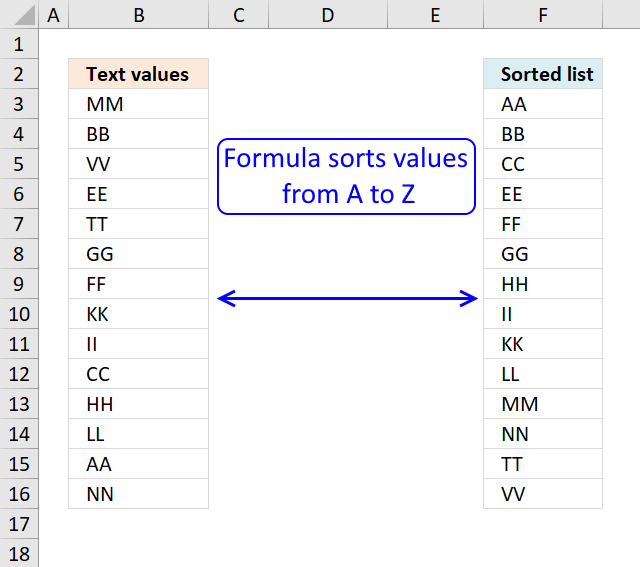Author: Oscar Cronquist Article last updated on October 31, 2018Table of Contents

I am inspired once again by the article Sorting Text in Excel using Formulas at Pointy haired Dilbert. In Chandoo´s article he sorts text with a "helper" column. My goal with this article is to show you how to sort text cells alphabetically without a helper column.

Here is an example, cell range \$A\$2:\$A\$15 contains text values.

Here is how to automatically sort text cells without any user interaction in cell B2:

Array formula in cell B2:

=INDEX(\$A\$2:\$A\$15, MATCH(SMALL(COUNTIF(\$A\$2:\$A\$15, "<"&\$A\$2:\$A\$15), ROW(1:1)), COUNTIF(\$A\$2:\$A\$15, "<"&\$A\$2:\$A\$15), 0))

Sort both numerical and text values with blank cells:

This article demonstrates how to filter a unique distinct list sorted from A to Z:

Create a unique distinct alphabetically sorted list

The array formula in cell D3 extracts unique distinct values sorted A to Z, from column B to column D. […]

The following post shows you how to sort and filter duplicate values alphabetically:

Extract a list of alphabetically sorted duplicates from a column

The following array formula extracts duplicate values sorted from A to Z from cell range B3:B21. Excel array formula in […]

Learn how to sort a multi-column cell range alphabetically and display the values in a single column:

Sort a range from A to Z [Array formula]

Question: How do I sort a range alphabetically using excel array formula? Answer: Cell range \$B\$2:\$E\$5 contains text values in random […]

Sort a data set using an array formula:

Sort a table with an array formula

The sorted table to the right is created with an array formula using the data in the table to the left. Array […]

Tip! You can sort a data set in any way you like it, if you convert it to an excel defined table: Excel table - Sort data

### How to create an array formula

1. Copy (Ctrl + c) and paste (Ctrl + v) array formula into formula bar.
2. Press and hold Ctrl + Shift.
3. Press Enter once.
4. Release all keys.

Copy cell B2 and paste it down as far as needed.

Recommended article

How to enter an array formula

Array formulas allows you to do advanced calculations not possible with regular formulas.

### Explaining array formula in cell B2

=INDEX(\$A\$2:\$A\$15, MATCH(SMALL(COUNTIF(\$A\$2:\$A\$15, "<"&\$A\$2:\$A\$15), ROW(1:1)), COUNTIF(\$A\$2:\$A\$15, "<"&\$A\$2:\$A\$15), 0))

Step 1 - Count "smaller" values

COUNTIF(range,criteria) counts the number of cells within a range that meet the given condition

=INDEX(\$A\$2:\$A\$15, MATCH(SMALL(COUNTIF(\$A\$2:\$A\$15, "<"&\$A\$2:\$A\$15), ROW(1:1)), COUNTIF(\$A\$2:\$A\$15, "<"&\$A\$2:\$A\$15), 0))

COUNTIF(\$A\$2:\$A\$15, "<"&\$A\$2:\$A\$15)

becomes

COUNTIF({MM,BB,VV,EE,TT,GG,FF,KK,KK,II,CC,HH,LL,AA,NN}, "<"&MM,"<"&BB,"<"&VV,"<"&EE,"<"&TT,"<"&GG,"<"&FF,"<"&KK,"<"&II,"<"&CC,"<"&HH,"<"&LL,"<"&AA,"<"&NN)

The first value in this array formula is "MM". Let´s see what happens when COUNTIF calculates how many values is small than "MM".

COUNTIF({MM,BB,VV,EE,TT,GG,FF,KK,II,CC,HH,LL,AA,NN}, "<"&"MM"

becomes

COUNTIF({MM"<"&"MM",BB"<"&"MM",VV"<"&"MM",EE"<"&"MM",TT"<"&"MM",GG"<"&"MM",FF"<"&"MM",KK"<"&"MM",II"<"&"MM",CC"<"&"MM",HH"<"&"MM",LL"<"&"MM",AA"<"&"MM",NN"<"&"MM"}

MM<MM is FALSE and BB<MM is TRUE and so on.. The array becomes:

COUNTIF({FALSE, TRUE, FALSE, TRUE, FALSE, TRUE, TRUE, TRUE, TRUE, TRUE, TRUE, TRUE, TRUE, FALSE}

becomes

COUNTIF({0, 1, 0, 1,0, 1 , 1 ,1 ,1 ,1 ,1 ,1 ,1 ,1 ,1 ,1 ,0} and the total is 10 which is the first number in the returning array. 0+1+0+1+0+1+1+1+1+1+1+1+1+1+1+1+0 = 10

Next is COUNTIF({MM,BB,VV,EE,TT,GG,FF,KK,II,CC,HH,LL,AA,NN}, "<"&"BB")

becomes

COUNTIF({MM"<"&"BB",BB"<"&"BB",VV"<"&"BB",EE"<"&"BB",TT"<"&"BB",GG"<"&"BB",FF"<"&"BB",KK"<"&"BB",II"<"&"BB",CC"<"&"BB",HH"<"&"BB",LL"<"&"BB",AA"<"&"BB",NN"<"&"BB"})

becomes

COUNTIF({FALSE, FALSE, FALSE, FALSE, FALSE, FALSE, FALSE, FALSE, FALSE, FALSE, FALSE,FALSE , TRUE, FALSE})

becomes

COUNTIF({0, 0, 0, 0,0, 0, 0,0,0,0,0,0,0,0,0,1,0}) and the total is 1 which is the second number in the returning array.

and so on...

COUNTIF(\$A\$2:\$A\$15, "<"&\$A\$2:\$A\$15) returns this array:

{10, 1,13,3,12,5,4,8,7,2,6,9,0,11}

Recommended article

How to use the COUNTIF function

Counts the number of cells that meet a specific condition.

Step 2 - Return the k-th smallest number in array

SMALL(array,k) returns the k-th smallest row number in this data set.

SMALL({10, 1,13,3,12,5,4,8,7,2,6,9,0,11}, ROW(1:1)) returns the smallest number in this data set, 0 (zero).

Recommended article

How to use the SMALL function

The SMALL function lets you extract a number in a cell range based on how small it is compared to the other numbers in the group.

Step 3 - Find position in array

MATCH(lookup_value;lookup_array; [match_type]) returns the relative position of an item in an array that matches a specified value

MATCH(SMALL(COUNTIF(\$A\$2:\$A\$15, "<"&\$A\$2:\$A\$15), ROW(1:1)), COUNTIF(\$A\$2:\$A\$15, "<"&\$A\$2:\$A\$15), 0)

becomes

MATCH(0, {10, 1,13,3,12,5,4,8,7,2,6,9,0,11}, 0) and returns 13.

Recommended article

How to use the MATCH function

Identify the position of a value in an array.

Step 4 - Return value

INDEX(array,row_num,[column_num]) returns a value or reference of the cell at the intersection of a particular row and column, in a given range.

=INDEX(\$A\$2:\$A\$15, MATCH(SMALL(COUNTIF(\$A\$2:\$A\$15, "<"&\$A\$2:\$A\$15), ROW(1:1)), COUNTIF(\$A\$2:\$A\$15, "<"&\$A\$2:\$A\$15), 0))

becomes

=INDEX(\$A\$2:\$A\$15, 13) and returns AA in cell B2.

Recommended article

How to use the INDEX function

Gets a value in a specific cell range based on a row and column number.

### Download excel sample file for this tutorial

Sorting-text-cells-using-array-formulas.xls
(Excel 97-2003 Workbook *.xls)

Recommended posts

Sort text cells alphabetically from two columns

Table of Contents Sort text from two columns combined (array formula) Sort text from multiple cell ranges combined (user defined […]

Populate drop down list with unique distinct values sorted from A to Z

Question: How do I create a drop-down list with unique distinct alphabetically sorted values? Table of contents Sort values using […]

Read more related articles

## Two columns sorting by the second columnArray Formula in cell E2:

=INDEX(\$B\$2:\$B\$9, MATCH(SMALL(COUNTIF(\$B\$2:\$B\$9, "<"&\$B\$2:\$B\$9), ROW(1:1)), COUNTIF(\$B\$2:\$B\$9, "<"&\$B\$2:\$B\$9), 0))

Array Formula in cell D2:

=INDEX(\$A\$2:\$A\$9, SMALL(IF(E2=\$B\$2:\$B\$9, MATCH(ROW(\$B\$2:\$B\$9), ROW(\$B\$2:\$B\$9))), SUM(--(E2=\$E\$2:E2))))

### Download excel *.xlsx

Two columns sorting by the second column.xlsx